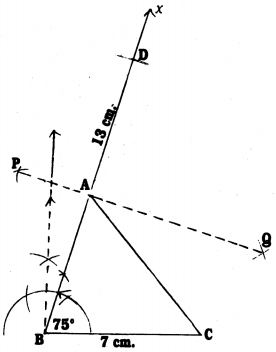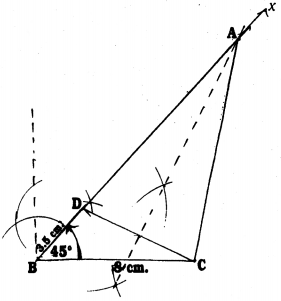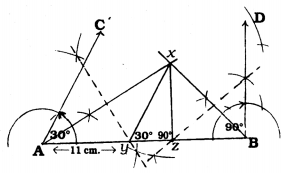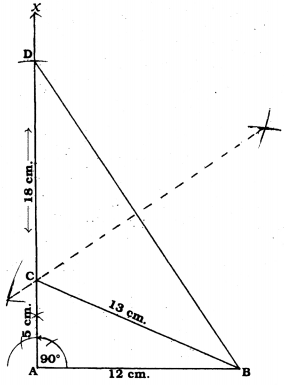# KSEEB Solutions for Class 9 Maths Chapter 6 Constructions Ex 6.2

KSEEB Solutions for Class 9 Maths Chapter 6 Constructions Ex 6.2 are part of KSEEB Solutions for Class 9 Maths. Here we have given Karnataka Board Class 9 Maths Chapter 6 Constructions Exercise 6.2.

## Karnataka Board Class 9 Maths Chapter 6 Constructions Ex 6.2

Question 1.
Construct a triangle ABC in which BC = 7 cm, ∠B = 75° and AB + AC = 13 cm,
Solution:
Construction of a triangle ABC in which BC = 7 cm, ∠B = 75° and AB + AC = 13 cmSteps of Construction :

1. Draw BC = 7 cm line segment.
2. Construct ∠B = 75° and produce it upto Bx.
3. With B as centre draw an arc BD = 13 cm such that it intersects Bx at D.
4. Construct PQ which is the perpendicular bisector of BD.
5. PQ intersects BD at A.
6. Join AC.
7. Now ∆ABC, with measurement AB + AC = 13 cm, and ∠B = 75°, BC = 7 cm is constructed.

Question 2.
Construct a triangle ABC in which BC = 8 cm, ∠B = 45° and AB – AC = 3.5 cm.
Solution:
Constructing a triangle ABC in which BC = 8 cm, ∠B = 45° and AB – AC = 3.5 cm.Steps of Construction :

1. Draw a line segment BC = 8 cm.
2. Draw ∠B = 45° with the help of a protractor. Bx is joined.
3. Draw an arc BD = 3.5 cm.
4. When perpendicular bisector DC is drawn, that intersects Bx at A. AC is joined.
5. Now, DABC with BC = 8 cm, ∠B = 45° and AB – AC = 3.5 cm. is constructed.

Question 3.
Construct a triangle PQR in which QR = 6 cm, ∠Q = 60° and PR – PQ = 2 cm.
Solution:
Constructing a triangle PQR in which QR = 6 cm, ∠Q = 60° and PR – PQ = 2 cm.Steps of Construction :

1. Draw a line segment QR = 6 cm.
2. With the help of a protractor draw a line segment Qx and ∠Q = 60°.
3. Produce line segment xQ upto y. mark T such that QT = 2 cm. Join TR.
4. If the perpendicular bisector of TR is drawn, that meets line segment Qx at P. PR is joined.
Now, ∆PQR is constructed.

Question 4.
Construct a triangle XYZ in which ∠Y = 30°, ∠Z = 90° and XY + YZ + ZX = 11 cm.
Solution:
Constructing a triangle XYZ in which ∠Y = 30°, ∠Z = 90° and XY + YZ + ZX = 11 cm.Steps of Construction :

1. Draw AB = 11 cm line segment with measurement XY + YZ + ZX.
2. With the help of protractor construct ∠A = 30°, ∠B = 90°.
3. Construct angular bisectors of ∠CAB and ∠DBA which meet at x. Ax, Bx are joined.
4. If perpendicular bisector AX is drawn, it intersects AB at Y and AB at Z.
5. By joining XY and XZ, ∆XYZ is constructed.

Question 5.
Construct a right triangle whose base is 12 cm and the sum of its hypotenuse and the other side is 18 cm.
Solution:
Constructing a right-angled triangle ABC with base, AB =12 cm and the sum of hypotenuse BC and another side AC, AC + BC = 18 cm.Steps of Construction :

1. Draw a line segment AB = 12 cm.
2. With the help of a protractor construct ∠A = 90° and produce upto AX.
3. Mark ‘D’ on AX such that AD = 18 cm.
4. Join BD.
5. Let the perpendicular bisector BD intersects AD at C. Join BC.
6. Now required triangle ABC is constructed with Base AB = 12cm, Side AC = – 5 cm, Hypotenuse BC = 13 cm and ∠A = 90°.

We hope the KSEEB Solutions for Class 9 Maths Chapter 6 Constructions Ex 6.2 help you. If you have any query regarding Karnataka Board Class 9 Maths Chapter 6 Constructions Exercise 6.2, drop a comment below and we will get back to you at the earliest.

error: Content is protected !!